## A tank in the shape of an inverted right circular cone has height 4 meters and radius 2 meters. It is filled with 3 meters of hot chocolate.

Question

A tank in the shape of an inverted right circular cone has height 4 meters and radius 2 meters. It is filled with 3 meters of hot chocolate. Find the work required to empty the tank by pumping the hot chocolate over the top of the tank. The density of hot chocolate is δ=1020 kg/m3.δ=1020 kg/m3. Your answer must include the correct units.

in progress 0
2 months 2021-08-02T07:55:59+00:00 1 Answers 3 views 0

The required work to empty the the tank is 247401 J.

Explanation:

Given that,

A tank is in the shape of right circular cone.

The radius of the tank= 2 m

The height of the tank = 4 m

The relation between the radius and the height is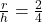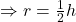Let h be the height of hot of chocolate any time t.

The volume of a cone is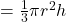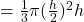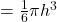The volume of the chocolate isThe mass of the  chocolate is(M)= Density × Volume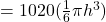Kg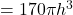kg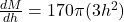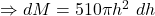Work done = Force × displacement

= Mass × acceleration×displacement

Here acceleration= acceleration due to gravity = 9.8 m/s²

The displacement when the hot chocolate level is h is = (4-h)

Work done (dw)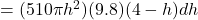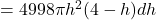Work done = W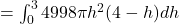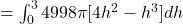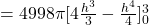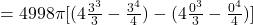=247401 J

The required work to empty the the tank is 247401 J.